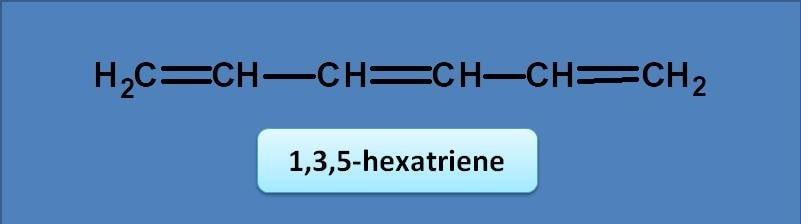# MCQ on UV-Visible spectroscopy: Page-12

1. Two compounds A and B have molar absorptivity as 1200 and 15,000 mol lit -1cm -1 respectively. Which of the following statements is correct regarding them?

(A) Compound A can be detected at very low concentrations than compound B.

(B) Compound B can be detected at very low concentrations than compound A.

(C) Both compounds can be detected at very dilute concentrations.

(D) Molar absorptivity have no influence on the detection of compounds.

2. Which of the following shows bathochromic shift in polar solvent

(A) Water

(B) Ethanol

(C) Ethylene

(D) Chloroform

Given below is 1,3,5-hexatriene. Calculate it’s l max .(A) 246 nm

(B) 245 nm

(C) 250 nm

(D) 247 nm

Normally diene has base value of 217 nm. Since the given compound is a triene, an extended conjugation produces an increment of 30 nm. Therefore the final value will be 217 + 30=247 nm.

4. The colour of a substance perceived by human eye is

(A) Same as that the substance emits in flame

(B) Same as that the substance absorbs

(C) Not same as that the substance absorbs

(D) Not same as that the substance emits

5. The color of potassium permanganate solution is due to

(A) n→π *

(B) π→π *

(C) d →d*

(D) n→s*